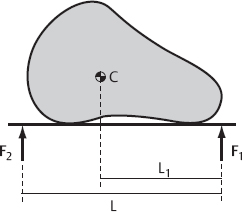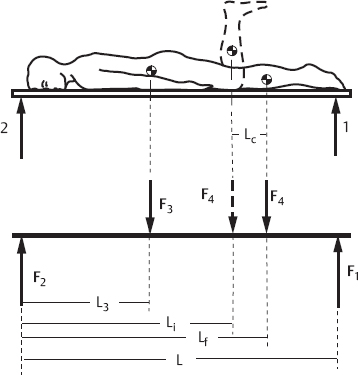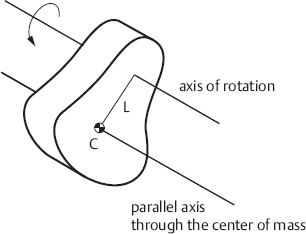# 11 Dimensions, Mass, Location of the Center of Mass, and Moment of Inertia of the Segments of the Human Body

10.1055/b-0036-140426

## 11 Dimensions, Mass, Location of the Center of Mass, and Moment of Inertia of the Segments of the Human Body

To describe the effect of gravity on the human body, and to derive moments and joint forces from observations of the moving body, the body is conceptualized as composed of individual segments connected by joints: for example, head, arm, trunk, thigh, lower leg, and foot. Depending on the problem under investigation, further subdivision may be appropriate. The arm, for example, can be subdivided into upper arm, lower arm, and hand. The body segments are conceptualized as rigid bodies, but this is only an approximation. In vivo the segments may change shape passively under external forces or actively through activation of the muscles. In addition, it is often impossible to define the boundary between segments precisely. Where exactly, for example, does the trunk end and the thigh begin?

Some reference values published in the scientific literature are based on measurements taken from only a small number of subjects. The reason is that some values have to be determined in vitro from autopsy specimens, which may not be available in large numbers. For this reason, the numbers cited below for the mass, dimension, location of the center of mass, and moment of inertia of body segments are not representative of both sexes and all age groups. They are to be regarded as estimates. They are approximately correct, but in the individual case deviations due to sex, age, and physique may occur. For simplicity, the reference values are often given not in absolute units but in relative units, per meter of height or per kilogram of body mass. This saves having to list values separately for persons of different height or body mass; however, individual variations in physique are ignored. Uncertainties in the values affect all investigations which use these data as input values, for example gait analysis. If high precision is required in the results, the individuals concerned have to be measured beforehand.

### 11.1 Determination of the Location of the Center of Mass and the Moment of Inertia

#### 11.1.1 Center of Mass

To describe the effect of a gravitational or inertial force on a body in a simple fashion, a point in space can be defined where the total mass m of the body is presumed to be concentrated. The gravitational or inertial force acts at this point, the so-called center of mass. (The term center of gravity is sometimes used as a synonym for center of mass. This is not strictly correct, since the centers of mass and gravity do not coincide if the gravitational force is different on different parts of a body, but such effects can be neglected in orthopedic biomechanics.) It must be pointed out that the center of mass is only a fictitious point; it does not have to coincide with an actual, material point in the body. The center of mass of an annulus, for example, is located at the center of the circle, a point where no mass is present.

A three-dimensional body can be subdivided into n small volume elements with masses mi (i 1, 2, ﻿… n). The gravitational or inertial force acts on each of these volume elements. Fig. 11.1 illustrates the calculation of the location of the center of mass using the example of a plane plate. For the purposes of calculation the plate is subdivided into n small quadratic elements with masses mi. The x- and y-coordinates of the center of mass Xc and Yc are calculated asFig. 11.1 Calculation of the location of the center of mass (C) of a plane plate. The plate is imagined as subdivided into small quadratic elements of masses mi. The coordinates of the center of mass in an (arbitrarily oriented) coordinate system are obtained by summation (or integration) extended over all mass elements multiplied by their distances from the axes and divided by the total mass of the plate.

where xi and yi are the coordinates of the elements with masses mi; m is the total mass of the plate. £ is the mathematical summation symbol and implies that the sum is to be extended over all masses mi from i = 1 to i = n. Explicitly, the formula for Xc reads

If the location of the center of mass of a three-dimensional body is to be calculated, we imagine the body as subdivided into small cubes. Xc and Yc are then calculated as given above. The z-coordinate Zc of the center of mass is obtained from

It can be shown (the proof is not given here) that the calculated location of the center of mass does not depend on the choice of xyz-coordinate system. Irrespective of the position and orientation of the coordinate system, the same physical location always results.

The segments of the human body have geometrically irregular shapes. In calculating the location of their center of mass, therefore, one may obtain an approximation by substituting simple geometric shapes. For example, the head could be modeled by a sphere or the lower arm by a cylinder, and a uniform density assumed. More refined models adjust the geometric shapes more closely to the body, and take account of density distributions within the segments (see, for example, Hatze 1).

Fig. 11.2 illustrates an experimental procedure for locating the center of mass of a plane plate. If the plate is suspended by a thread attached to an arbitrary point P on its rim, one knows that in static equilibrium its center of mass is located perpendicularly below point P. (If this were not the case, the plate would oscillate back and forth and a resting position would not be attained.) If the plate is suspended from two different points, the location of the center of mass is given by the intersection of the perpendiculars through the suspension points. The center of mass of a three-dimensional body can be obtained by suspending the body from three noncollinear points (points that are not in a line).Fig. 11.2 Experimental determination of the location of the center of mass of a plane plate. The plate is suspended by a thread. In static equilibrium the magnitude F of the tensile force of the thread and the magnitude m · g of the gravitational force of the plate are opposite and equal; the center of mass is located below the suspension point P, somewhere along the dashed line. Suspending the plate from a second point allows the center of mass to be located.

Alternatively, the location of the center of mass can be measured from the support reactions of a two-point support, each support instrumented with a force transducer ( Fig. 11.3 ). In equilibrium of moments, force transducer 1 on the right-hand side of the setup measures the force asFig. 11.3 Experimental determination of the location of the center of mass by measuring the support reaction on a two-point support, each support instrumented with a force transducer. If one reaction force, the mass of the object, and the distance between the supports are known, the distance L1 of the center of mass from the support can be calculated.

This equation is obtained by summing all moments in relation to support 2 on the left-hand side. In the formula m designates the mass of the body, g is the gravitational acceleration, L is the distance between the supports, and L1 is the distance of the center of mass from support 1. By solving the equation for L1, the distance of the center of mass from support 1 is obtained. To obtain the other two coordinates of the center of mass, the measurement is carried out twice more with the body rotated by 90° each time.

If the mass of a segment of the human body is known, it is possible to determine the location of its center of mass in vivo. Fig. 11.4 illustrates the procedure for the example of the segment consisting of the lower leg and foot. The body is positioned on a base supported by two force transducers. The mass of the lower leg and foot is designated by m. The magnitude of force F 1 on transducer 1 is measured in the initial state i (knee flexed 90°) and in the final state f (knee extended). Related to the point of application of force F 2, the sums of the moments (all forces designated by their magnitudes) readFig. 11.4 Example of in vivo determination of the location of the center of mass of a body segment. If the mass of the lower leg and foot is known, the location of the center of mass of this body segment can be calculated from the support reactions measured with the knee bent at 90° (initial state i) and with the knee extended (final state f). Alternatively, if the location of the center of mass is known, the mass of the segment can be obtained.

Subtraction results in

The distance Lc from the center of mass of the lower leg to the knee’s axis of rotation is given by

If the location of the center of mass is known, the method can also be employed to determine the mass of the segment. To do this, Eq. 11.7 has to be solved for m.

#### 11.1.2 Moment of Inertia

A point mass at distance L from an axis of rotation has the moment of inertia I

The moment of inertia has the dimension [kg · m2]. A three-dimensional body can be imagined as subdivided into small volume elements, each contributing to the total moment of inertia. If the body is subdivided into n cube-shaped elements with masses mi ( Fig. 11.5 ), the moment of inertia is calculated asFig. 11.5 To calculate the moment of inertia, the body is imagined as composed of small volume elements of mass mi. The moment of inertia is obtained by summation over all masses mi multiplied by the square of their distance Li from the axis of rotation.

where Li designates the distance of the i-th volume element from the axis of rotation. The sum is to be extended over all volume elements. If the mass is continuously distributed within the volume, Eq. 11.9 is written in form of an integral to be extended over the whole volume V:

It follows from the definition that a moment of inertia is always related to a specific axis of rotation. If the axis is changed, the moment of inertia changes as well. Since the number of axes is unlimited, an unlimited number of moments of inertia also exist. If, however, one confines the discussion to axes running through the center of mass, this multiplicity of axes can be reduced to three principal moments of inertia. The principal moments are moments in relation to three mutually perpendicular axes running through the center of mass: the principal axes. It can be shown (the proof is not given here) that the moment of inertia about any axis that runs through the center of mass can be calculated if the three principal moments are known. For bodies such as spheres, cylinders, or cuboids, which are symmetrical in relation to certain axes, the principal axes coincide with the axes of symmetry. As an example, Fig. 11.6 shows the direction of the principal axes and the respective principal moments of inertia, obtained by integration, for a sphere and a cylinder with uniform mass distribution.Fig. 11.6 Coordinate systems employed for specifying the moments of inertia of a solid cylinder and solid sphere of uniform density ρ. These simple geometric shapes can be used as models for body segments.

For a cylinder of density ρ, mass m, and moments of inertia Ix, Iy, Iz about the x-, y-, and z-axes, it holds that

For a solid sphere of density ρ mass m, and moments of inertia Ix, Iy, Iz about the x-, y-, and z-axes, it holds that

Further examples of principal axes and moments for geometrically simple shapes are given in mechanics textbooks and formula collections.

If the moment of inertia of a body in relation to an axis through its center of mass is known, the moment in relation to any other axis parallel to this axis can easily be obtained. The parallel axis theorem states that “the moment of inertia about an arbitrary axis is equal to the moment about a parallel axis running through the center of mass plus the moment of the total mass m, concentrated at the center of mass, about the arbitrary axis” ( Fig. 11.7 ).Fig. 11.7 Parallel axis theorem. The moment of inertia about an arbitrary axis is equal to the moment Ic about a parallel axis through the center of mass plus the moment of inertia m · L2, where m is the mass of the body and L the distance of the center of mass from the axis of rotation.

where I denotes the moment of inertia about the arbitrary axis, Ic the moment about the parallel axis through the center of mass, m the mass of the body, and L the distance of the axis from the center of mass.

As we have said, the segments of the human body (head, trunk, thigh, lower leg, etc.) are irregularly shaped. To calculate approximate values of their moments of inertia, the true shapes may be replaced by simple geometric shapes of comparable dimensions, for example the head by a sphere or the lower arm by a cylinder. A refined model may substitute an ellipsoid for the head, a hemisphere for the shoulder, or a truncated cone for the thigh. Another requirement for finer modeling is knowledge of the density distribution. Knowledge of the density distribution within human body segments is incomplete, but as an approximation, a uniform density between that of bone and soft tissue may be chosen.

Alternatively, the moment of inertia of a body about a given axis can be obtained experimentally by setting up the body to swing about that axis and observing it. In the setup shown in Fig. 11.7 , the body oscillates about an axis located at a distance L from the center of mass. The angular frequency of an undamped physical pendulum (see textbooks of physics) is given by

where ω is the observed angular frequency, T the oscillation period, m the mass of the body, g the gravitational acceleration, L the distance of the axis from the center of mass, I the moment of inertia about the axis of oscillation, and Ic the moment about a parallel axis through the center of mass. If the mass of the body and the location of its center of mass in relation to the axis of oscillation are known and the oscillation period T is measured, the moments I and Ic can be calculated.

If in the setup shown in Fig. 11.7 the axis of rotation were to pass through the center of mass (L = zero), the pendulum would not oscillate. If, however, the body is suspended from a torsion spring, for example from a thin elastic wire, which deforms in torsion, any deflection from the idle state will generate a moment. The body will then execute a rotary oscillation with a frequency

where D denotes the torsion coefficient of the torsion spring and Ic the moment of inertia related to an axis which passes through the center of mass. By using the measured period of oscillation T, the moment of inertia Ic can be calculated. (In this experiment, the torsion coefficient D of the torsion spring is determined beforehand by observation of the oscillation of a body with a known moment of inertia.) Moments of inertia of segments of the human body have been determined in vitro using both the physical pendulum and the torsional pendulum method. In vivo, too, the moments of inertia of an arm or a leg have been measured by observing free oscillations of these body segments about anatomical joint axes (physical pendulum method). However, such experiments require that the muscles crossing the joints should be completely relaxed and that the surrounding soft tissue should not damp the oscillation too much.

Moments of inertia of segments of the human body (head, arms, trunk, etc.) are usually not cited in units of kilograms per square meter [kg · m2]. Instead, the radius of gyration i is given in meters [m]. From the radius of gyration i and the mass ms of the segment, the moment of inertia I can be calculated as

Stating the radius of gyration has the advantage that the variations in body segment weight can be accounted for in a simple fashion. This presumes that body segments of persons of differing body mass are geometrically similar. In reality this is only approximately true—think, for example, of the differences in the shape of the thigh in different individuals. Occasionally, the relative radius of gyration irel is stated; this is equal to the radius of gyration divided by the length of the segment. Stating the relative radius of gyration has the advantage that, for every body segment, a single number suffices to quantify the moment of inertia in persons of differing body height.